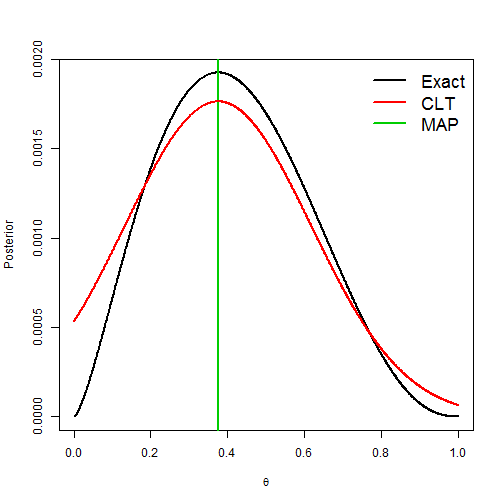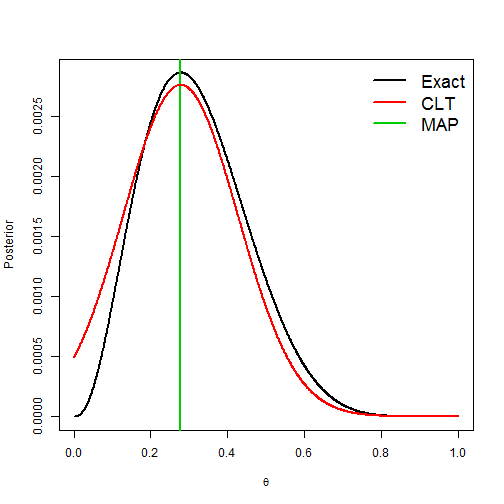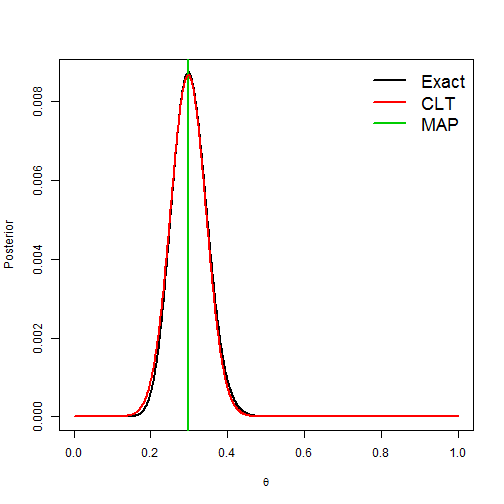# Bayesian Central Limit Theorem Approximation

### Chapter 3.1.3: Bayesian Central Limit Theorem (CLT)

The model is $$Y|\theta\sim\mbox{Binomial}(n,\theta)$$ and $$\theta\sim\mbox{Beta}(½,½)$$. The exact posterior is $$\theta|Y\sim\mbox{Beta}(Y+½,n+½)$$. Below we compare the exact posterior with a Gaussian approximation to the posterior for small, medium, and large datasets (as determined by $$n$$).

The Gaussian approximation is centered around the maximum a posteriori (MAP) estimate $${\hat \theta} = A/(A+B)$$ where $$A=Y-½$$ and $$B=n-Y-½$$. This is found by taking the derivative of the log posterior with respect to $$\theta$$, setting it to zero, and solving for $$\theta$$. The posterior variance is approximated as $$\frac{1}{\frac{A}{{\hat \theta}^2}+\frac{B}{(1-{\hat \theta})^2}}$$. This is a function of the second derivative of the log posterior evaluated at $${\hat \theta}$$.

## Approximation in a small sample case

 theta <- seq(0.001,0.999,.001) # Grid of thetas for plotting
Y     <- 2                     # The data
n     <- 5

# Compute the posterior mean and Fisher information matrix

A         <- Y-0.5
B         <- n-Y-0.5
theta_MAP <- A/(A+B)
Info      <- A/theta_MAP^2+B/(1-theta_MAP)^2

# Plot the true and approximate posteriors

post1 <- dbinom(Y,n,theta)*dbeta(theta,0.5,0.5)
post1 <- post1/sum(post1)
post2 <- dnorm(theta,theta_MAP,sqrt(1/Info))
post2 <- post2/sum(post2)

plot(theta,post1,type="l",lwd=2,
xlab=expression(theta),ylab="Posterior")
abline(v=theta_MAP,col=3,lwd=2)
lines(theta,post2,col=2,lwd=2)
legend("topright",c("Exact","CLT","MAP"),bty="n",col=1:3,cex=1.5,lwd=2)## Approximation in a medium sample case

 theta <- seq(0.001,0.999,.001) # Grid of thetas for plotting
Y     <- 3                     # The data
n     <- 10

# Compute the posterior mean and Fisher information matrix

A         <- Y-0.5
B         <- n-Y-0.5
theta_MAP <- A/(A+B)
Info      <- A/theta_MAP^2+B/(1-theta_MAP)^2

# Plot the true and approximate posteriors

post1 <- dbinom(Y,n,theta)*dbeta(theta,0.5,0.5)
post1 <- post1/sum(post1)
post2 <- dnorm(theta,theta_MAP,sqrt(1/Info))
post2 <- post2/sum(post2)

plot(theta,post1,type="l",lwd=2,
xlab=expression(theta),ylab="Posterior")
abline(v=theta_MAP,col=3,lwd=2)
lines(theta,post2,col=2,lwd=2)
legend("topright",c("Exact","CLT","MAP"),bty="n",col=1:3,cex=1.5,lwd=2)## Approximation in a large sample case

 theta <- seq(0.001,0.999,.001) # Grid of thetas for plotting
Y     <- 30                    # The data
n     <- 100

# Compute the posterior mean and Fisher information matrix

A         <- Y-0.5
B         <- n-Y-0.5
theta_MAP <- A/(A+B)
Info      <- A/theta_MAP^2+B/(1-theta_MAP)^2

# Plot the true and approximate posteriors

post1 <- dbinom(Y,n,theta)*dbeta(theta,0.5,0.5)
post1 <- post1/sum(post1)
post2 <- dnorm(theta,theta_MAP,sqrt(1/Info))
post2 <- post2/sum(post2)

plot(theta,post1,type="l",lwd=2,
xlab=expression(theta),ylab="Posterior")
abline(v=theta_MAP,col=3,lwd=2)
lines(theta,post2,col=2,lwd=2)
legend("topright",c("Exact","CLT","MAP"),bty="n",col=1:3,cex=1.5,lwd=2)